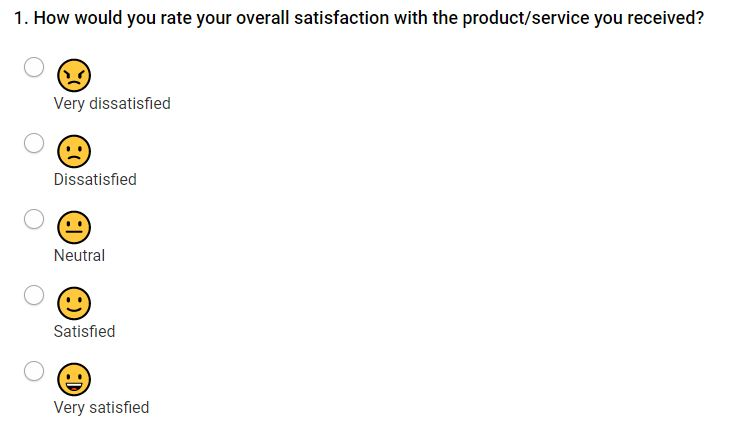# Satisfaction Rate Calculations

"Satisfaction Rate" is calculated for Customer Satisfaction Score questions. It's calculated to give you an overall percentage of how satisfied your respondents are with your products and services.When each respondent answers this type of question, it is totalled within the results. When you view the results for this particular question you will be able to see how many respondents chose each answer choice.

By using this information, the satisfaction rate can be calculated.

Because the rate is automatically calculated for you, you should never have to perform this calculation yourself; the purpose of this article is to "show the working" of the calculation so the metric can be properly understood.

The satisfaction rate is calculated in the following steps:

### Apply a score to each answer option.

Each answer choice, or column heading, within the satisfaction question is assigned a value starting from 0, which increases depending on the number of options.

In single answer questions, all of the answer choices are counted. In matrix questions, only the number of columns are counted)

For the example question given at the top of the article:

• Very Dissatisfied: 0
• Dissatisfied: 1
• Neutral: 2
• Satisfied: 3
• Very Satisfied: 4

### Determine the "Maximum Possible Value".

We add together the total number of responses for the question - here is an example set of answer data for our question.This total is then multiplied by the maximum value for the question's answer choices or column headings.
In this example, the maximum value is 4.

The total of this equation gives us the Maximum Possible Value. This is the value we would have if all our respondents had chosen the highest level of satisfaction.

261 x 4 = 1044

### Calculate the 'Actual Total Value'.

To do this, the total number of times that each answer choice was selected is multiplied by the value assigned to that answer choice.• Very Dissatisfied: 0 x 6 = 0
• Dissatisfied: 1 x 9 = 9
• Neutral: 2 x 25 = 50
• Satisfied: 3 x 145 = 435
• Very Satisfied: 4 x 76 = 304

The resulting total for each answer choice or column heading is then added together. This will give us the Actual Total Value.

0 + 9 + 50 + 435 + 304 = 798

### The final step is to calculate the 'Satisfaction Rate'.

By dividing the Actual Total Value with the Maximum Possible Value and then multiplying by 100 we get a percentage Value.

(798 / 1044) x 100 = 76.44%

So, that's our satisfaction rate.